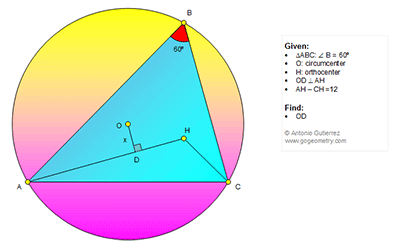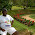## Thursday, February 2, 2017

### Geometry Problem 1312: Triangle, 60 Degrees, Circle, Circumcenter, Orthocenter, Altitude, Distance, Perpendicular, Measurement

Geometry Problem. Post your solution in the comment box below.
Level: Mathematics Education, High School, Honors Geometry, College.

Details: Click on the figure below.1.Problem 1312
The extension of OH intersects AB and BC in K,L respectively .According to the problem 761 the triangle BKL is equilateral .Is <AOC=2<ABC=120, <AHC=90+<ABC/2=120.So
AOHC is cyclic.Draw CPM//KL (M in AB, P in AH).Is <AMC=120=<AOC or AMOHC is
, so AH-HC=AH-HP=AP=12.Draw MN perpendicular AP. Is OD=MN=x, MA=2x and
triangle MAN from pythagorean theorem 4x^2=x^2+36 or x=2√(3 ).
APOSTOLIS MANOLOUDIS KORYDALLOS PIRAEUS GREECE

2.Extend AH to meet BC at E
The perpendicular from O to BC cuts BC in half and say meet at P.
So OPED is a rectangle with PE = OD = x -------(1)
let AB = c and consider the 30-60-90 triangle ABE
we have BE = c/2 and AE = Sqrt(3)c/2 -------(2)
From (1) and (2) EC = PC-PE = BP-PE = BE-PE-PE = c-4x/2 --------(3)
Now consider the 30-60-90 triangle ECH
we have
HE = (c-4x)/2Sqrt(3)
CH = (c-4x)/Sqrt(3) --------(4)

Given AH-CH = 12
=> (AE-HE)-CH = 12
=> Sqrt(3)c/2-(c-4x)/2Sqrt(3)- (c-4x)/Sqrt(3) = 12 (from (2) and (4))
=> 3c-c+8x-2c+4x = 12*2*Sqrt(3)
=> x = 2Sqrt(3)

3.Let R be the circumradius.

Since < AOC = < AHC = 120, AOHC is concyclic.
So < OHD = 30 and OH = 2x

Applying Ptolemy,
2x.b + R. CH = R. AH

But R = b/sqrt3 considering Tr. AOC.

So x = (AH - CH).R/b = (12 / 2)/sqrt3
x = 2.sqrt3

Sumith Peiris
Moratuwa
Sri Lanka

4.Extend AH to P (P on circle) => ΔHPC equilateral
Extend CH to M (M on circle) => ΔABC ≡ ΔAMC so if we draw perpendicular to
CM and AB we get x inradius of AHG (G on AB)
AD = 1/2 AP => AD = DH+HP = DH+HC (1) But AH-HC = 12 (2)
From (1) and (2) DH = 6
From ΔODH and pythagore theorem x = 2√3

5.https://goo.gl/photos/Mo9Wudh9iSaMpX4S8

Let c= AH, a= HC
Let AH cut circle ABC at E
Note that BC is the perpen. Bisector of HE => HCE is equilateral
So HE=HC=a
D is the midpoint of AE so AD= ½(a+c)
AOK is 30-60-90 triangle so AO= AC/sqrt(3)
In triangle AHC we have angle AHC= 120 and AC^2= a^2+c^2+a.c
In triangle AOD => x^2= AO^2-AD^2
X^2= 1/3(a^2+c^2+a.c)-1/4(a+c)^2
After simplification we get 12.x^2= (a-c)^2= 144
So x=2.sqrt(3)

6.Sumith Peiris, Moratuwa, Sri Lanka
- This solution is EXCELLENT!

1.